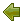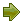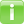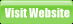Project
Code
Meter

[article cited from Wikipedia]

Halstead complexity measures are software metrics introduced by Maurice Howard Halstead in 1977. These metrics are computed statically, without program execution.

### Calculation

First we need to compute the following numbers, given the program source code:

• n1 = the number of distinct operators
• n2 = the number of distinct operands
• N1 = the total number of operators
• N2 = the total number of operands

From these numbers, five measures can be calculated:

• Program length: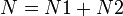$N = N1 + N2 \,$
• Program vocabulary: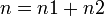$n = n1 + n2 \,$
• Volume: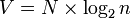$V= N \times \log_2 n \,$
• Difficulty :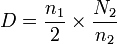$D= { n_1 \over 2 } \times { N_2 \over n_2 } \,$
• Effort: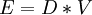$E= D * V \,$

The difficulty measure is related to the difficulty of the program to write or understand, e.g. when doing code review.